Time Value of Money - Six Functions of a Dollar
Using Assessors Handbook Section 505 (Capitalization Formulas and Tables)
Appraisal Training: Self-Paced Online Learning Session

# Lesson 6: Present Worth of \$1 Per Period(Assessors’ Handbook 505, Column 5)

This lesson discusses the Present Worth of \$1 Per Period (PW\$1/P); one of six compound interest functions presented in Assessors’ Handbook Section 505 (AH 505), Capitalization Formulas and Tables. The lesson:

• Explains the function’s meaning and purpose,
• Provides the formula for the calculation of PW\$1/P factors, and
• Shows practical examples of how to apply the PW\$1/P factor.

## PW\$1/P: Meaning and Purpose

The PW\$1/P is the present value of a series of future periodic payments of \$1, discounted at periodic interest rate i over n periods, assuming the payments occur at the end of each period. The PW\$1/P is typically used to discount a future level income stream to its present value.

Another way to conceptualize the PW\$1/P is the amount that must be deposited today to fund withdrawals of \$1 at the end of each of the n periods at periodic interest rate i, assuming a periodic rate i can be earned on the outstanding balance.

This compound interest function, together with the PW\$1, is the basis of yield capitalization and its primary variant, discounted cash flow analysis. The PW\$1/P factors are in column 5 of AH 505.

## Formula for Calculating PW\$1/P Factors

The formula for the calculation of the PW\$1/P factors is as follows: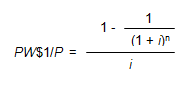Where:

• PW\$1/P = Present Worth of \$1 Per Period Factor
• i = Periodic Interest Rate, often expressed as an annual percentage rate
• n = Number of Periods, often expressed in years

In order to calculate the PW\$1/P factor for 4 years at an annual interest rate of 6%, use the formula below: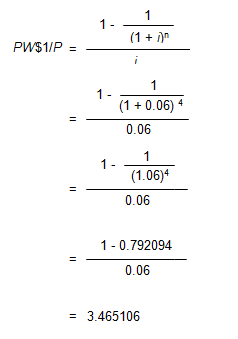Viewed on a timeline: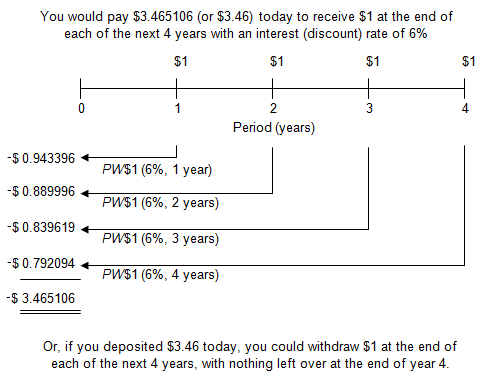On the timeline, the initial deposit of \$3.465106 is shown as negative because from the point of view of a depositor it would be a cash outflow. The future values of \$1 at the end of each year are shown as positive because they would be cash inflows.

To locate the PW\$1/P factor, go to page 33 of AH 505, go down 4 years and across to column 5. The correct factor is 3.465106.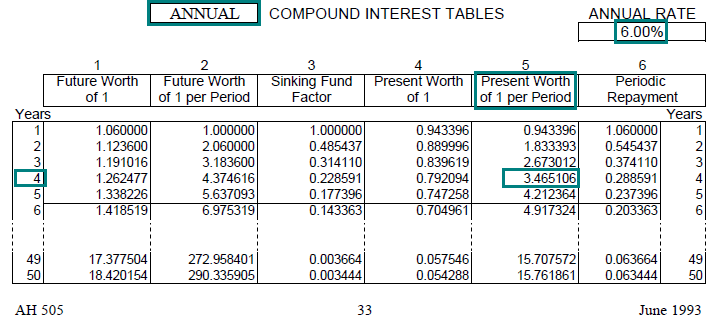Link to AH 505, page 33

## Practical Applications of PW\$1/P

Example 1:
You’ve admired your neighbor’s vintage car for years, and he’s finally agreed to sell it to you. He offers you the following payment alternatives:

1. Pay \$20,000 now, or
2. Pay \$6,000 at the end of each of the next 4 years with an annual interest rate of 8%

Which is the better alternative?

Solution:

• PV = PMT × PW\$1/P (8%, 4 years, annual)
• PV = \$6,000 × 3.312127
• PV = \$19,873

Calculate the present value of the 4-year payment plan (alternative 2) using the PW\$1/P factor and compare it to the immediate payment of \$20,000 (alternative 1).

• Find the annual PW\$1/P factor (annual compounding) for 8% at a term of 4 years. In AH 505, page 41, go down 4 years and across to column 5 to find the correct factor of 3.312127.
• The present value of \$19,873 is equal to the periodic payment of \$6,000 multiplied by the factor.
• You want to select the payment alternative with the lowest cost in present-value terms. Because the present value of the four payments (\$19,873) is less than the immediate payment of \$20,000 (no discounting of the immediate payment is required), the four-payment alternative is preferable after adjusting for the time value of money.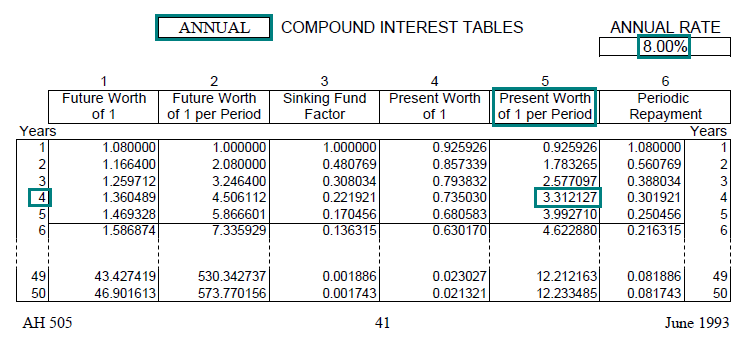Link to AH 505, page 41

Example 2:
You will receive annual payments of \$10,000 at the end of each year for the next 15 years with an annual interest rate of 5%. What is the present value of this stream of payments?

Solution:

• PV = PMT × PW\$1/P (5%, 15 years, annual)
• PV = \$10,000 × 10.379658
• PV = \$103,796.58
• Find the annual PW\$1/P factor (annual compounding) for 5% and 15 years. In AH 505, page 29, go down 15 years and across to column 5 to find the correct factor of 10.379658.
• The present value of \$103,796.58 is equal to the periodic payment of \$10,000 multiplied by the factor.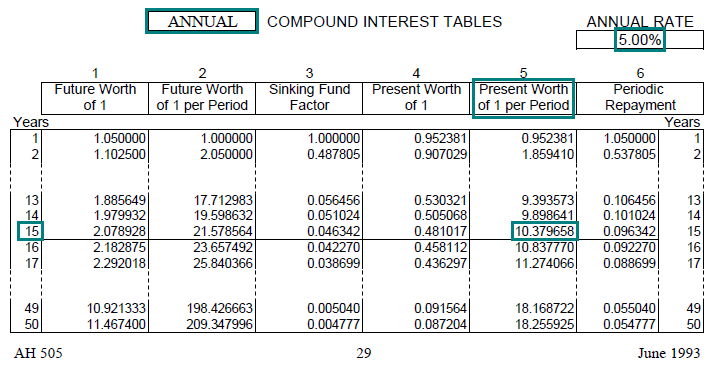Link to AH 505, page 29

Example 3:
At the end of each year following your retirement, you want to withdraw \$20,000 from your 401k retirement account. You expect to live for 20 years after you retire. Assuming that you can earn an annual interest rate of 6%, what balance will you need in your retirement account to fund your planned withdrawals?

Solution:

• PV = PMT × PW\$1/P (6%, 20 years, annual)
• PV = \$20,000 × 11.469921
• PV = \$229,398
• Find the annual PW\$1/P factor (annual compounding) for 6% and 20 years. In AH 505, page 33, go down 20 years and across to column 5 to find the correct factor of 11.469921.
• The present value of \$229,398 is equal to the annual payment of \$20,000 multiplied by the PW\$1/P factor. When you retire, the balance in your account must equal the present value of the 20 years of planned future withdrawals.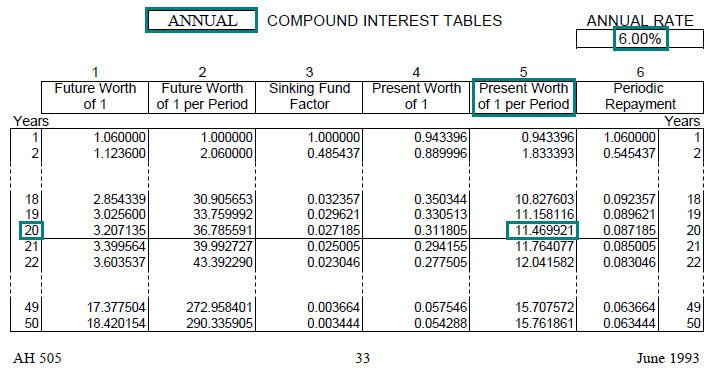Link to AH 505, page 33

Example 4:
Mr. Fortunate has won the \$64 million California lottery. He will receive 20 annual payments of \$3,200,000, with the first payment to be received immediately. Acme Investment Company is offering Mr. Fortunate \$30,000,000 for the right to receive his 20 payments. If the annual interest rate is 8% with annual compounding, should he accept the offer?

Solution:

• PV = PMT × PW\$1/P (8%, 19 years, annual)
• PV = \$3,200,000 × 9.603599
• PV = \$30,731,517

• Total PV = \$30,731,517 + \$3,200,000 = \$33,931,517
• Use the PW\$1/P factor for 19 years to discount the future 19 payments of \$3,200,000 (AH 505, page 41, column 5).
• Add the initial payment of \$3,200,000 (this occurs immediately and is not discounted) to calculate the total present value of the promised payments.
• Acme is offering \$30,000,000 for a stream of cash flows valued at \$33,931,517 (assuming an 8% discount rate). Mr. Fortunate should decline Acme’s offer.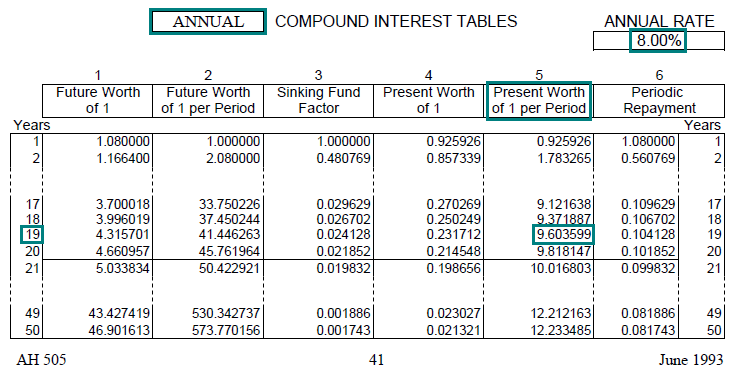Link to AH 505, page 41

Example 5:
The subscription to your favorite magazine is about to expire. The magazine company offers you three renewal options:

1. Pay \$100 now for a four-year subscription.
2. Pay \$32 per year at the end of each year for four years.
3. Pay \$54 today and another \$54 two years from today.

Assuming you want to receive the magazine for at least four more years, if the annual interest rate is 10%, which option is the best deal?

Solution:

• PV = PMT × PW\$1/P (10%, 4 years, annual)
• PV = \$32 × 3.169865
• PV = \$101.44
•
• PV = FV × PW\$1 (10%, 2 years, annual)
• PV = \$54.00 × 0.826446
• PV = \$44.63
• Total PV = \$54.00 + \$44.63 = \$98.63
• Determine the present value of each renewal option and select the option with the lowest present value.
• The present value of option 1 is \$100; payment is immediate and no discounting is required.
• The present value of option 2 is calculated using the PW\$1/P factor (AH 505, page 49, column 5).
• The present value of option 3 is the initial payment of \$54 (no discounting required) plus the present value of the second payment of \$54 discounted for 2 years using the PW\$1 factor (AH 505, page 49, column 4). Option 3 has the lowest present value and is the best deal.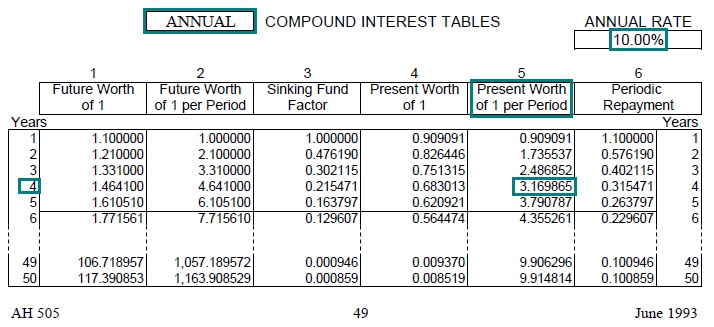Link to AH 505, page 49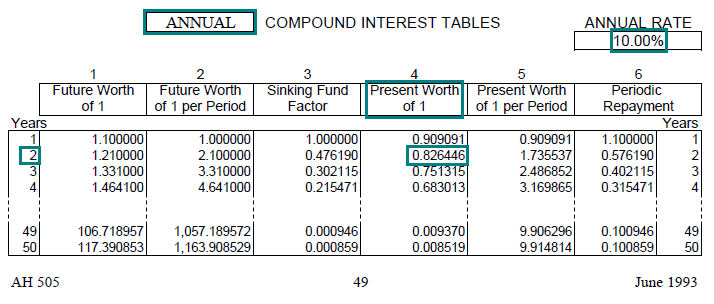Link to AH 505, page 49